### Load Distribution Three Wheeled Vehicle

3. Load Distribution Three Wheeled Vehicle

The forces acting on a vehicle at rest are shown in figure.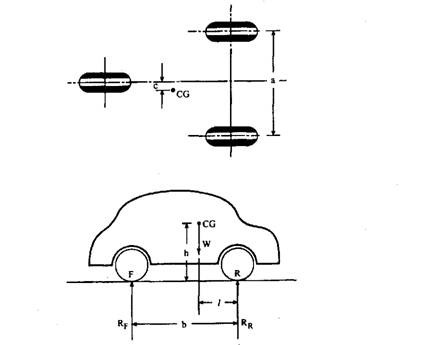Where,

W= weight of the wheel, N,

b= wheelbase, m,

l=distance of CG from the rear axle, m,

h=height of CG from road surface, m,

c=distance of CG from the central axis, m,

a= wheel track, m, RF = vertical reaction at front wheel, N,

RR1, RR2 = vertical reaction at the rear wheels,

N There are three unknowns which can be determined as follows:

Moment about rear axle gives Therefore moment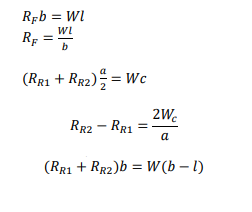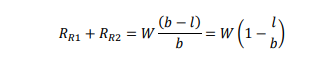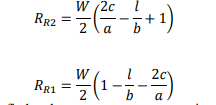Also W=RF+RR1+RR2 must be satisfied and server as an extra equation for alternative solution

4. FOUR-WHEELED VEHICLE

Forces acting on a four-wheeled vehicle at rest, we can form three independent equations to take care of four unknown viz., four reactions at the wheels. Thus the problem is simplified by considering it as a two-wheeled vehicle, i.e. the reactions on both rear wheels are equal and also on both front wheels. Let RF and RR be vertical reactions at front and rear wheel respectively, The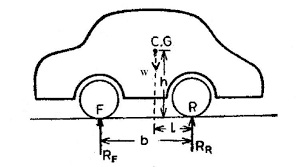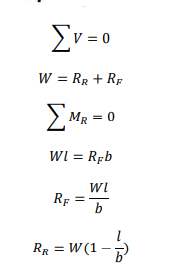Substituting the value of RR in the ABOVE EQUATION